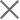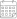Subscribe

### Indexes

Quarter 1-2, 2022 | Energy Market Review24 October 2022

In the first and the second quarters of 2022, Georgian power plants generated 3,107 mln. and 3,663 mln. kWh of electricity, respectively. This represents a 27.9% and 35.3% increase in total generation compared to the corresponding periods of the previous year (in 2021, the total generation in Q1 was 2,429 mln. kWh and 2,708 mln. kWh in Q2). On an annual basis, the increase in generation in the first quarter of 2022 was derived from a 26.1% and 30.1% increase in thermal and hydropower generation, respectively, more than offsetting the 5.2% decrease in wind power generation. The increase in production in the second quarter is related to the 120.2% and 27.3% increase in thermal and hydropower generation, more than offsetting the 12% decrease in wind power generation.

In the first and second quarters of 2022, electricity consumption on the local market amounted to 3,920 mln. (Q1) and 3,660 mln. kWh (Q2) (+13% and +9.9% compared to the first and second quarters of 2021, respectively) (Figure 1). Total power consumption exceeded generation by 813 mln.kWh in the first quarter of the year and generation exceeded consumption in the second by 4 mln.kWh. In percentage terms, in Q1 there was a generation deficit amounting to 26.2% of total generation and in Q2 there was a generation surplus of 0.1% of total generation, respectively. In 2021, total consumption exceeded generation in the first quarter by 1040 mln. kWh, around 42.8% of the total generation, and the difference between total generation and consumption resulted in a shortage in the second quarter of 623 mln. kWh, around 18.7% of the total generation.